#1

## 【小馬的資結演算法秘笈】(3)堆積排序法- heapSort

heap這個單字中文翻譯叫作堆，

heap是一個二元樹的結構，滿足兩個性質:

• heap property: 每個父節點要比小孩節點還要小(滿足此特性為min heap，若父節點比小孩節點還要大則為 max heap)
• shape property: 指heap的長相一定要由上到下、由左到右的擺放節點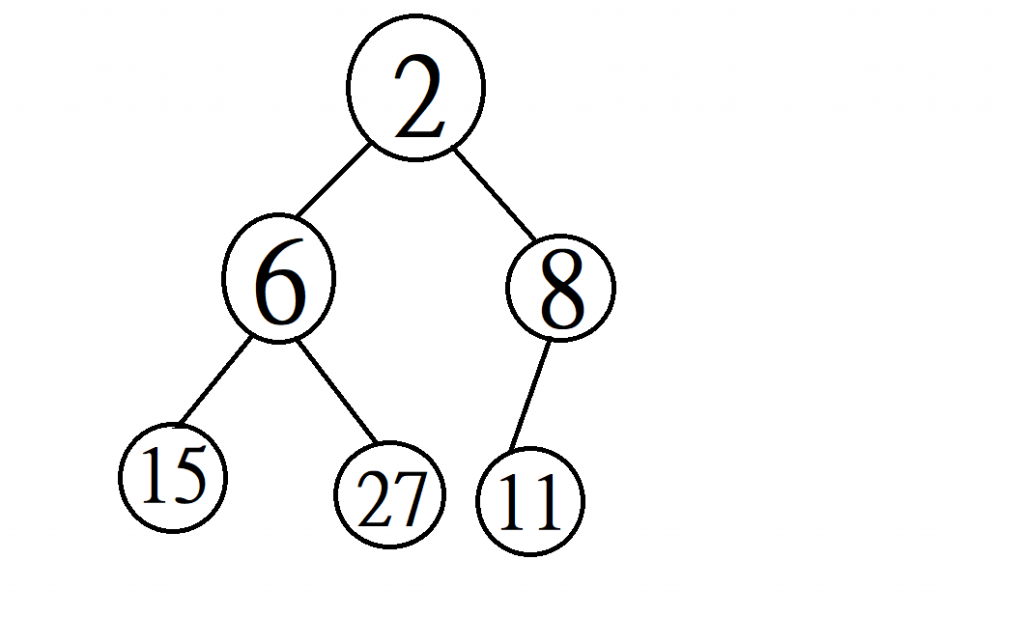insert: 在heap中插入一個元素
extract-min: 把heap中最小的元素拿走

# insert- O(log n)的時間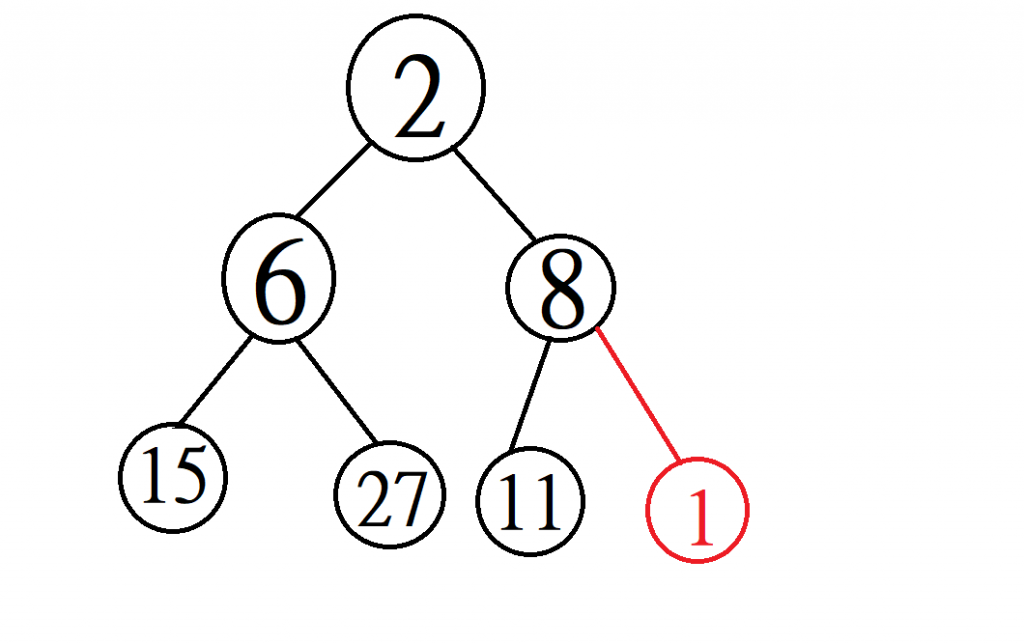heap property說上面的點一定要比下面的點還要小，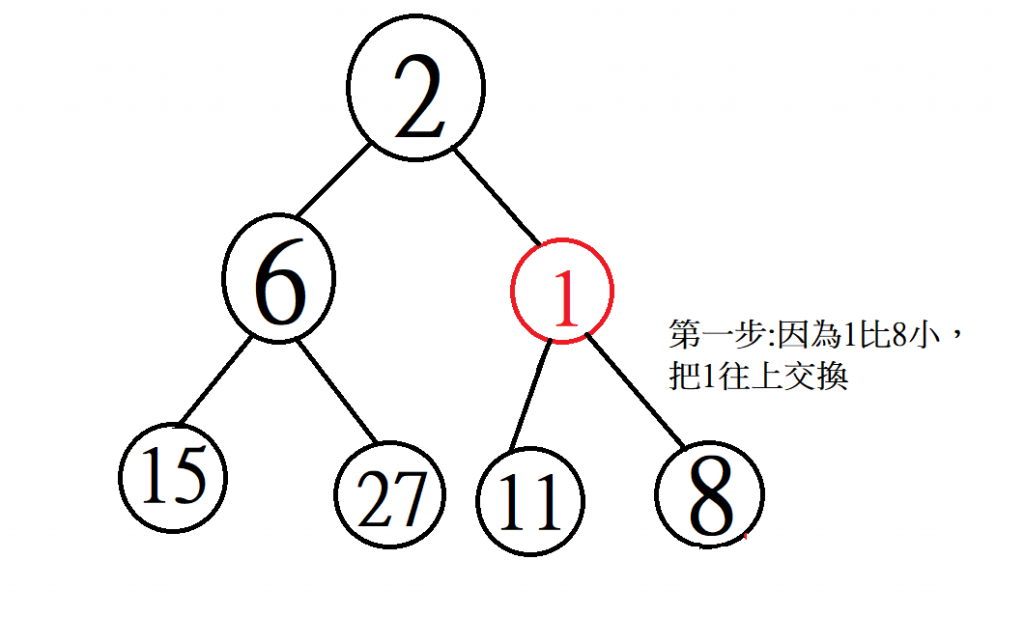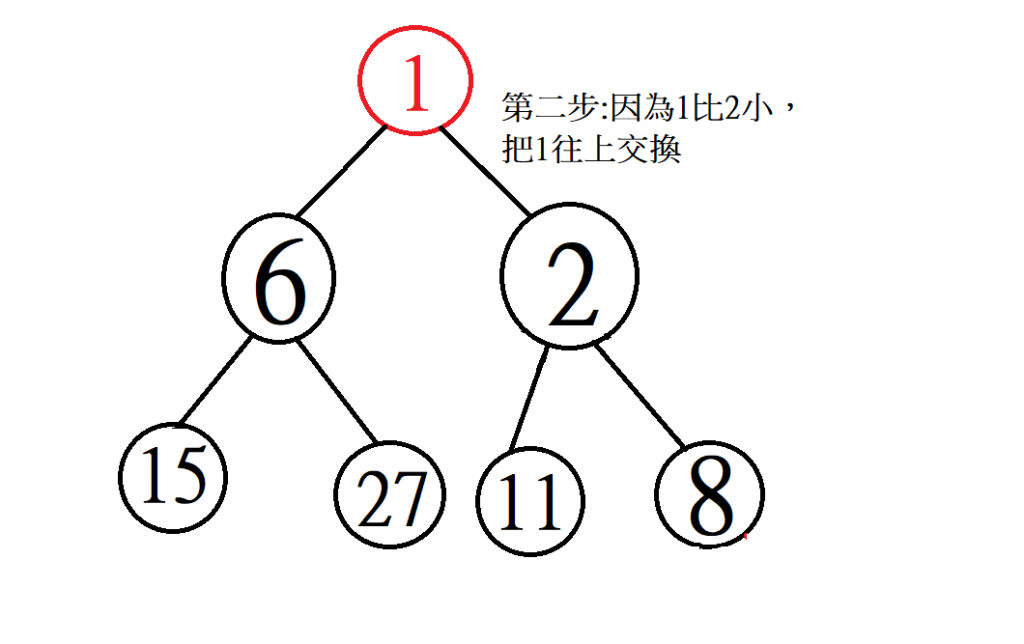# extract-min- O(log n)的時間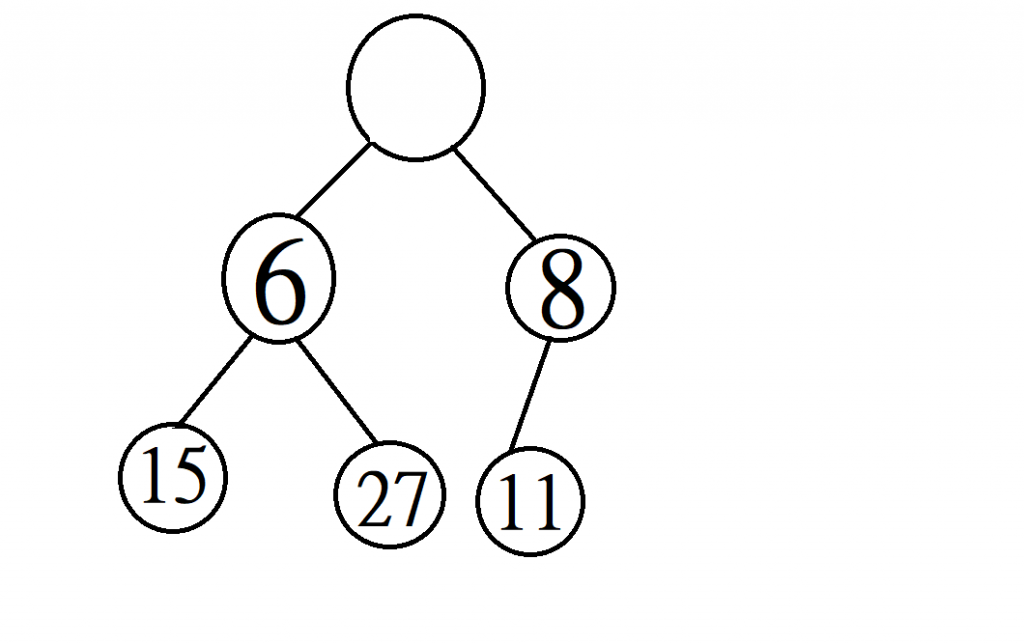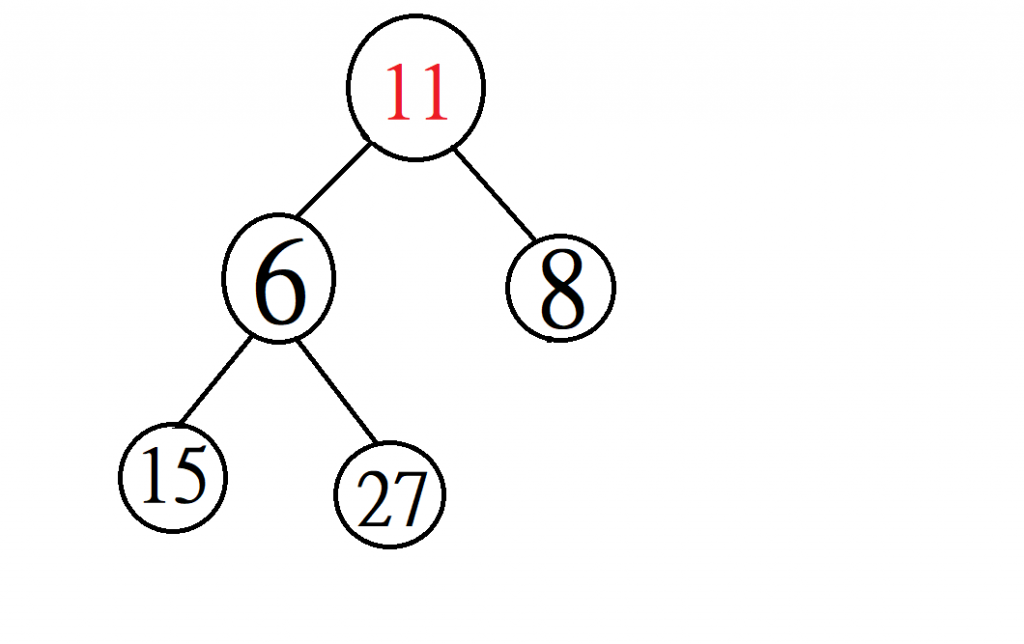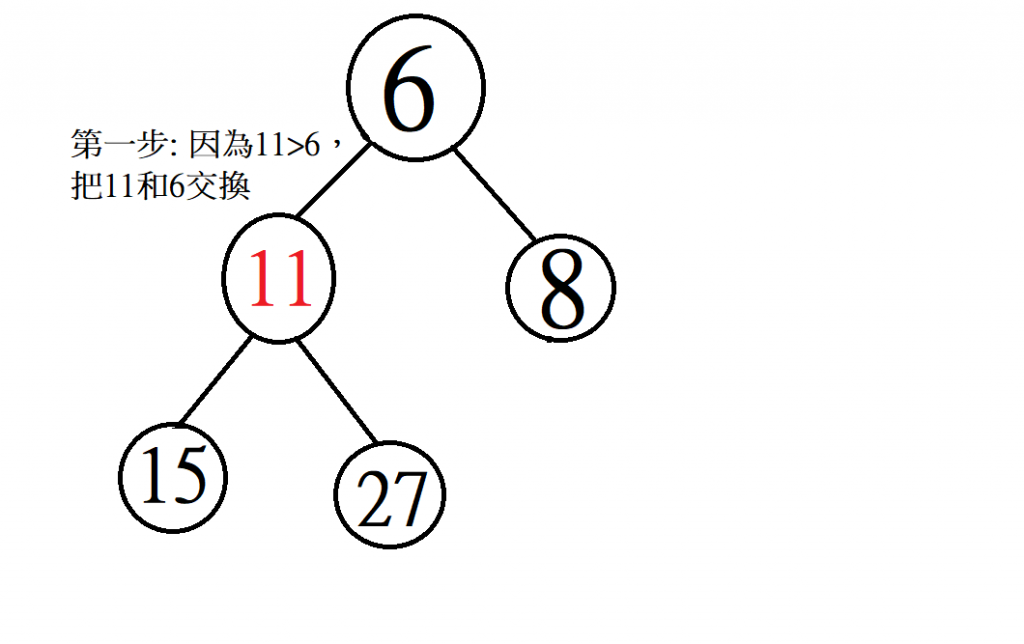(注意不能把11跟8交換，不然8會比6還大)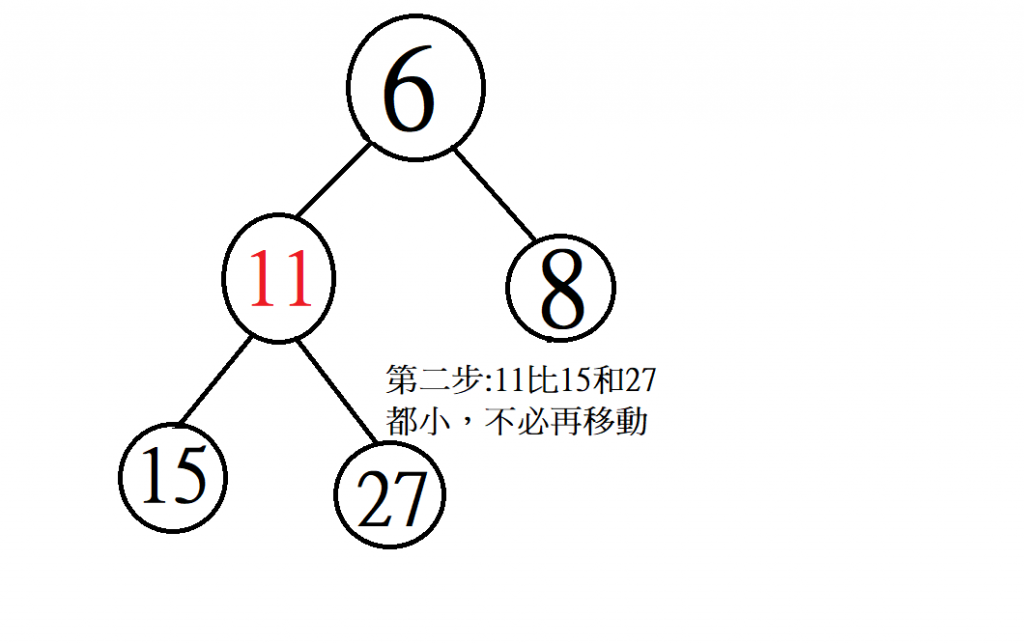# 程式如何存一個heap?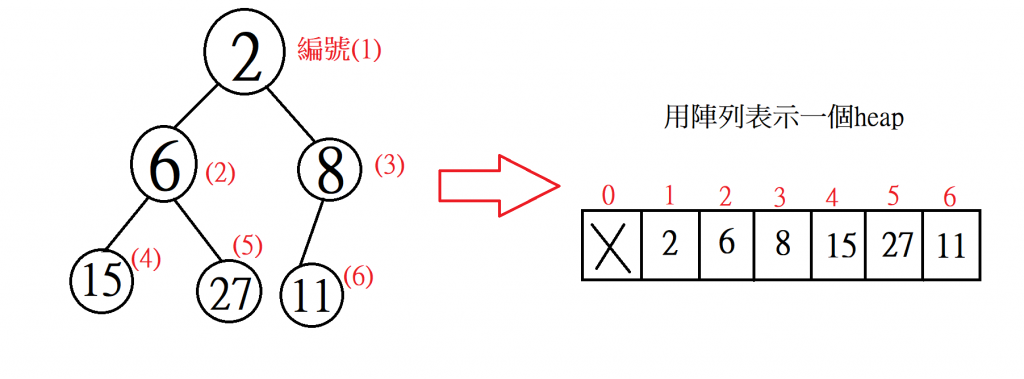• 父節點的編號是「i/2」(尾數捨去)
• 小孩節點的編號分別是「2*i」與「2*i+1」

# 如何用heap幫助我們排序

1. 先不斷的用insert函數把元素放到heap裡面 (共插入n個元素，每次要log n的時間)
2. 在不斷用extract-min幫我們把元素從heap裡面拿出來 (共拿出n個元素，每次要log n的時間)

# 程式碼範例

``````#include <iostream>
#include <vector>
using namespace std;

class Heap {
private:
vector<int> array = {0}; //初始給一個元素，讓index0的位置閒置
public:
Heap() = default;
Heap(vector<int> _array);
void insert(int num);
int extract_min();
vector<int> HeapSort();
};

Heap::Heap(vector<int> _array) {
for (int i = 0; i < _array.size(); i++) {
insert(_array[i]);
}
}

void Heap::insert(int num) {
array.push_back(num);
int idx = array.size()-1;
while (idx / 2 > 0 and array[idx] < array[idx / 2]) {
swap(array[idx], array[idx / 2]);
idx /= 2;
}
}

int Heap::extract_min() {
int min_element = array;
int sz = array.size()-1; //元素個數
swap(array, array[sz]);
int idx = 1;
while (idx * 2 <sz) {
int small_chlid_idx = idx * 2; //判斷小孩節點比較小的那個編號
if (small_chlid_idx + 1 < sz && array[small_chlid_idx + 1] < array[small_chlid_idx])
small_chlid_idx++;
if(array[idx] > array[small_chlid_idx])
swap(array[idx], array[small_chlid_idx]);
idx = small_chlid_idx;
}
array.erase(array.end()-1); //清掉最後一個元素(即最小的那個)
return min_element;
}

vector<int> Heap::HeapSort() {
int sz = array.size() - 1; //元素個數
vector<int> vec;
for (int i = 0; i < sz; i++) {
vec.push_back(extract_min());
}
return vec;
}

int main()
{
vector<int> vec = { 1,5,6,3,2,8,10,7 };
Heap heap(vec);
vec = heap.HeapSort();
for (int i = 0; i < vec.size(); i++)
cout << vec[i] << " " ;
cout << endl;
return 0;
}
``````